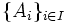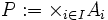# Box topology

## Contents

View a complete list of basic definitions in topology

## Definition

Let$I$ be an indexing set, and$\{ A_i \}_{i \in I}$ be a family of topological spaces. Define:$P := \times_{i \in I} A_i$

to be the Cartesian product of the$A_i$s. The box topology is a topology on$P$ in terms of the topologies on$A_i$s defined in the following equivalent ways:

1. It is a topology with basis as the open boxes or open rectangles: sets of the form$\times_{i \in I} U_i$ where each$U_i$ is open in$A_i$
2. Given a basis for each space$A_i$, it is a topology with basis as sets of the form$\times_{i \in I} U_i$, where each$U_i$ is a basis element of$A_i$

### Equivalence of definitions

Further information: Equivalence of definitions of box topology, Open boxes satisfy the condition for a basis

## Related notions

Product topology is a related, and more useful, topology on the Cartesian product of topological spaces. In fact, the default topology endowed on the Cartesian product of topological spaces is the product topology. The box topology and product topology are equal for products of only finitely many spaces. For infinite products, the product topology is a coarser topology, because it admits in its basis only those open rectangles where all but finitely many of the open sides are the whole space.

A list of properties of topological spaces closed under taking box products is available at Category:Properties of topological spaces closed under box products.